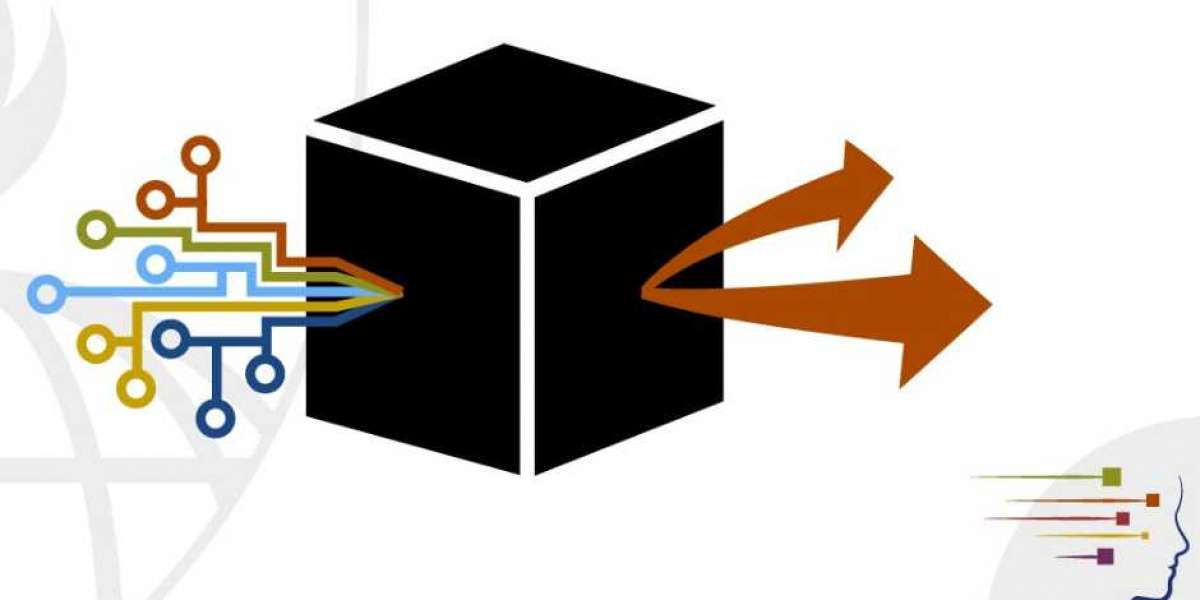## FEATURE ENGINEERING TECHNIQUES OVER NUMERICAL AND CATEGORICAL FEATURES FOR MACHINE LEARNING.

Feature engineering is a vital part of this. Without this step, the accuracy of your machine learning algorithm reduces significantly. A typical machine learning starts with data collection and exploratory analysis. Data cleaning comes next. This step removes duplicate values and correctin

It is very important to attest how crucial feature engineering can be. Feature engineering has immense potential, but it can be slow and arduous process when done manually.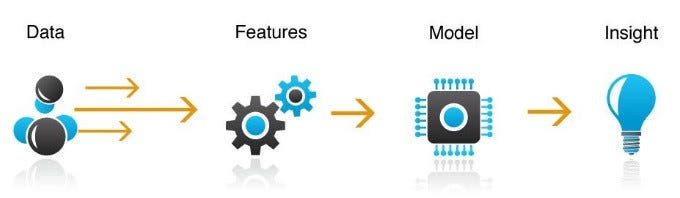What is feature engineering?

Feature engineering is the process of transforming raw data into features that better represent underlying problem to predictive models resulting in improved accuracy of unseen data.

Importance of feature engineering:

• The features in data will directly influence the predictive models you use and the results that you can achieve.
• You can say that better the features you prepare the better the results you achieve.
• The results you achieve are a factor of model you choose and features you prepared.
• The flexibility of good features will allow you to use the less complex models that are faster to run and easier to understand and maintain.
• With good features you are closer to underlying problem and representation of all data you have and use to characterize underlying problem.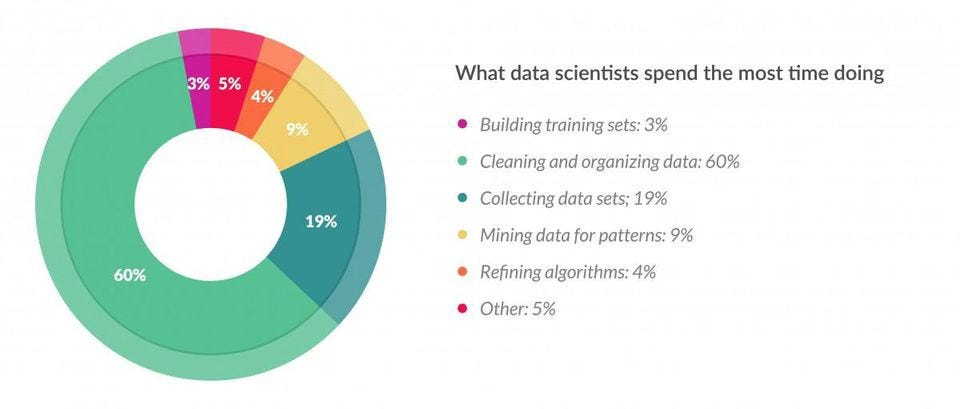Few common strategies that we use in machine learning:

1. IMPUTATION:

Missing values are one of common problems that occur when you deal with data. The missing values can occur due to the various reasons when obtaining the data.And missing the values also can be used when you perform the feature engineering on top of that data.

For example, when you are dealing with the medical data the test sample missing from the particular country can indicate that performing that particular sample test in that country may be costly or we can extract feature based on the missing data.

It is not good idea to always drop the data when the missing values are present.Because this can lead to the information loss or can create the imbalance in the data set . Using various strategies and hacks we can impute the missing data in the dataset.Imputation for numerical features:-

We can design several strategies to fill the null values or we can fill them using the suggestions of domain expert of that particular data type.

In case of numerical data it is always preferred to fill the values with median or mean. In fact median is better than mean because the mean can cause deviation.we can also fill the null values with the previous values or fill with the mathematical operation of other values based on the analyzing the problem statement and suggestions from the domain expert.

//"filling with null values"//
data['column_name']=data['column_name'].fillna(0)

//"filling with median values"//
data['column_name']=data['column_name'].fillna(data.median)

Imputation for categorical features:-

when we are dealing with the categorical features the concatenation of the other features or replacing with the frequently occurred value is preferable.

//"replacing with the frequently occured"//
data['column_name']=data['column_name'].fillna(data['column_name'].value_counts().idxmax(),inplace=True)

2.HANDLING OUTLIERS

Generally, the detection of outliers is done in the phase of exploratory data analysis.But it is not just the detection of outliers in case of machine learning but also how to handle the outliers and use them in the development and improvement of model.

For the detection of outliers, it is important to visualize them generally using the box plots, violin plots, scatter plots, percentiles, and value of z-score. Handling outliers is an important technique otherwise the patterns in the data get misinterpreted by the model.Outlier detection using the box plot-

import seaborn as sns
sns.boxplot(x=boston_df['DIS'])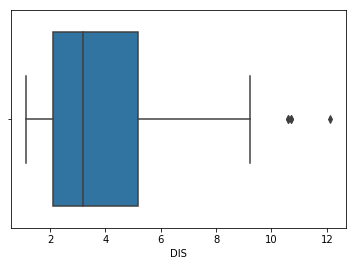boxplot for distance in BostonDataset

The above plot shows three points from 10 to 12 these are outliers that are not included in the box anywhere near the quartiles. In the above plot, we have done the analysis for the uni-variate outlier. we can do the analysis for the multivariate also when you have many categorical values occurring in the single feature column.

Scatter-plot:-

A scatter plot is a type of mathematical diagram using the cartesian coordinates to display values for typically two variables of a set of data. The data is displayed as a collection of points. Having the value of one variable determining the position on the horizontal axis and the value of another variable on the vertical axis.

fig, ax = plt.subplots(figsize=(16,8))
ax.scatter(boston_df['INDUS'], boston_df['TAX'])
ax.set_xlabel('Proportion of non-retail business acres per town')
ax.set_ylabel('Full-value property-tax rate per \$10,000')
plt.show()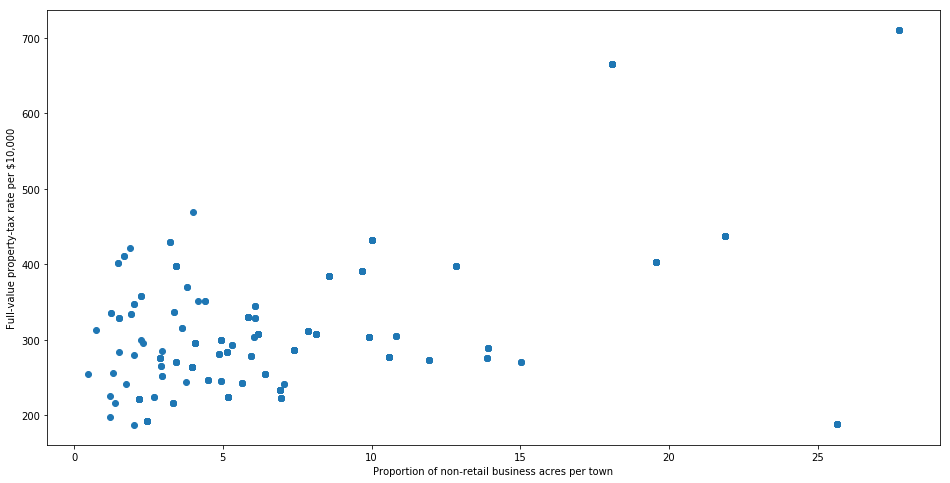visualizing the plot above we can see the left portion of the plot is denser with a more number of points than the right portion of the plot.

Outlier detection with percentiles:-

Outliers can be detected using the percentiles. we can setup the threshold value to decide whether the point is an outlier or not. we can set the minimum and maximum threshold values if the value is less than the minimum threshold or it is greater than the maximum threshold it can be declared as an outlier.

//Dropping the outlier rows with Percentiles//

upper_lim = data['column'].quantile(.95)
lower_lim = data['column'].quantile(.05)
data = data[(data['column'] upper_lim) (data['column'] lower_lim)]

we can detect the outliers and can handle them based on the dropping r capping the outliers. dropping is done based on the size of data and information loss that can occur. capping can be done based on the values of the threshold.

3.BINNING:-

Binning can be applied on both categorical and numerical features. It is very important method in feature engineering.//Numerical Binning Example//

Value      Bin
0-30   -  Low
31-70  -  Mid
71-100 -  High

//Categorical Binning Example//

Value      Bin
Spain  -  Europe
Italy  -  Europe
Chile  -  South America
Brazil -  South America

Binning is done to make the model more robust and to avoid overfitting. The labels with low frequencies probably affect the robustness of statistical models negatively.

//Numerical Binning Example//

data['bin'] = pd.cut(data['value'], bins=[0,30,70,100], labels=["Low", "Mid", "High"])

value   bin
0      2   Low
1     45   Mid
2      7   Low
3     85  High
4     28   Low

//Categorical Binning Example//

Country
0      Spain
1      Chile
2      Australia
3      Italy
4      Brazil

conditions = [
data['Country'].str.contains('Spain'),
data['Country'].str.contains('Italy'),
data['Country'].str.contains('Chile'),
data['Country'].str.contains('Brazil')]

choices = ['Europe', 'Europe', 'South America', 'South America']

data['Continent'] = np.select(conditions, choices, default='Other')

Country      Continent
0      Spain         Europe
1      Chile  South America
2  Australia          Other
3      Italy         Europe
4     Brazil  South America

4.LOG-TRANSFORM

Logarithmic transform is mostly used mathematical transformation in feature engineering.

The advantages of using the log transform are:-

• It helps to handle the skewed data after transformation.
• It decreases the effect of outliers due to the normalization of the magnitude differences model becomes more robust.

The data we apply log transform must have only positive values otherwise we get an error. we can add 1 to data to transform it.

//Log Transform Example//

data = pd.DataFrame({'value':[2,45, -23, 85, 28, 2, 35, -12]})data['log+1'] = (data['value']+1).transform(np.log)

//Negative Values Handling//
/Note that the values are different/

data['log'] = (data['value']-data['value'].min()+1) .transform(np.log)

 value log(x+1) log(x-min(x)+1) 0 2 1.09861 3.25810 1 45 3.82864 4.23411 2 -23 nan 0.00000 3 85 4.45435 4.69135 4 28 3.36730 3.95124 5 2 1.09861 3.25810 6 35 3.58352 4.07754 7 -12 nan 2.48491

5.ONE-HOT ENCODING AND LABEL ENCODING:-

One-hot encoding is one of the mostly used encoding methods. This method spreads values and assigns 0 or 1 to them. This method changes categorical data to understand our algorithm to numerical format.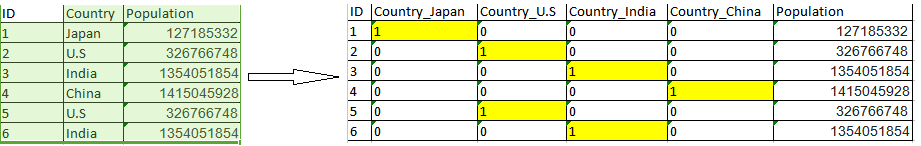One-hot encoding can lead to a multiple number of columns . When the column contains the less number of variation in the categorical variables then we can label encode them such that variants of same kind get encoded to the same number.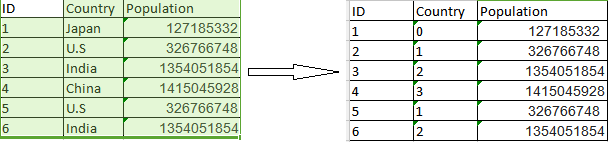LabelEncoding over Country feature

6.FEATURE SPLIT AND FEATURE MERGE:-Splitting the features and merging features is good way to make useful in terms of machine learning.Merging features can be done when the particular row has null data .we can merge the data present in the row to fill the null values in the row.

Suppose in case of personal cancer diagnosis data set the features gene,variation and text.gene is the feature related to the gene of the sample.variation is related to the type of the variation of the sample.Text is the description about the sample. when the text is null we can merge the features of gene and variation.Generally these are done from the suggestions from the domain expert.

Features that can be splitted and can be added as the new feature.All this we do if it increases the performance of the model.

data.name

0  Luther N. Gonzalez
1    Charles M. Young
2        Terry Lawson
3       Kristen White
4      Thomas Logsdon

//Extracting first names

data.name.str.split(" ").map(lambda x: x)

0     Luther
1    Charles
2      Terry
3    Kristen
4     Thomas

//Extracting last names

data.name.str.split(" ").map(lambda x: x[-1])

0    Gonzalez
1       Young
2      Lawson
3       White
4     Logsdon

CONCLUSION:-

The above strategies can give us an idea about feature engineering on discrete, categorical data. But while performing the feature engineering it is all about how we understand the problem statement and use the data perform the feature engineering on it for increasing the performance if the model.we have to remember that “garbage in, garbage out” in machine learning because it is all about features obtained from data.

Search
Categories